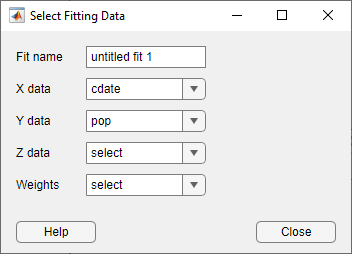Documentation

## Data Selection

### Selecting Data to Fit in Curve Fitting App

To select data to fit, use the drop-down lists in the Curve Fitting app to select variables in your MATLAB® workspace.

• To fit curves:

• Select X data and Y data.

• Select only Y data to plot `Y` against index (`x=1:length( y )`).

• To fit surfaces, select X data, Y data and Z data.

You can use the Curve Fitting app drop-down lists to select any numeric variables (with more than one element) in your MATLAB workspace.

Similarly, you can select any numeric data in your workspace to use as Weights.For curves, X, Y, and Weights must be matrices with the same number of elements.

For surfaces, X, Y, and Z must be either:

• Matrices with the same number of elements

• Data in the form of a table

For surfaces, weights must have the same number of elements as Z.

When you select variables, the Curve Fitting app immediately creates a curve or surface fit with the default settings. If you want to avoid time-consuming refitting for large data sets, you can turn off Auto fit by clearing the check box.

### Note

The Curve Fitting app uses a snapshot of the data you select. Subsequent workspace changes to the data have no effect on your fits. To update your fit data from the workspace, first change the variable selection, and then reselect the variable with the drop-down controls.

### Selecting Compatible Size Surface Data

For surface data, in Curve Fitting app you can select either Matrices of the Same Size or Table Data.

#### Matrices of the Same Size

Curve Fitting app expects inputs to be the same size. If the sizes are different but the number of elements are the same, then the tool reshapes the inputs to create a fit and displays a warning in the Results pane. The warning indicates a possible problem with your selected data.

#### Table Data

Table data means that `X` and `Y` represent the row and column headers of a table (sometimes called breakpoints) and the values in the table are the values of the `Z` output.

Sizes are compatible if:

• X is a vector of length `n`.

• Y is a vector of length `m`.

• Z is a 2D matrix of size `[m,n]`.

The following table shows an example of data in the form of a table with `n = 4 `and `m = 3`.

`x(1)``x(2)``x(3)``x(4)`
`y(1)``z(1,1)``z(1,2)``z(1,3)``z(1,4)`
`y(2)``z(2,1)``z(2,2)``z(2,3)``z(2,4)`
`y(3)``z(3,1)``z(3,2)``z(3,3)``z(3,4)`

Like the `surf` function, the Curve Fitting app expects inputs where `length(X) = n`, ```length(Y) = m``` and `size(Z) = [m,n]`. If the size of `Z` is `[n,m]`, the tool creates a fit but first transposes `Z` and warns about transforming your data. You see a warning in the Results pane like the following example:

```Using X Input for rows and Y Input for columns to match Z Output matrix.```

For suitable example table data, run the following code:

``` x = linspace( 0, 1, 7 ); y = linspace( 0, 1, 9 ).'; z = bsxfun( @franke, x, y ); ```

For surface fitting at the command line with the `fit` function, use the `prepareSurfaceData` function if your data is in table form.

#### Weights

If you specify surface Weights, assign an input the same size as Z. If the sizes are different but the number of elements is the same, Curve Fitting app reshapes the weights and displays a warning.

### Troubleshooting Data Problems

If there are problems with the data you select, you see messages in the Results pane. For example, the Curve Fitting app ignores `Inf`s, `NaN`s, and imaginary components of complex numbers in the data, and you see messages in the Results pane in these cases.

If you see warnings about reshaping your data or incompatible sizes, read Selecting Compatible Size Surface Data for information.

If you see the following warning: ```Duplicate x-y data points detected: using average of the z values```, this means that there are two or more data points where the input values (x, y) are the same or very close together. The default interpolant fit type needs to calculate a unique value at that point. You do not need do anything to fix the problem, this warning is just for your information. The Curve Fitting app automatically takes the average z value of any group of points with the same x-y values.

Other problems with your selected data can produce the following error:

```Error computing Delaunay triangulation. Please try again with different data.```
Some arrangements of data make it impossible for Curve Fitting app to compute a Delaunay triangulation. Three out of the four surface interpolation methods (linear, cubic, and nearest) require a Delaunay triangulation of the data. An example of data that can cause this error is a case where all the data lies on a straight line in x-y. In this case, Curve Fitting app cannot fit a surface to the data. You need to provide more data in order to fit a surface.

### Note

Data selection is disabled if you are in debug mode. Exit debug mode to change data selections.